# Generate random numbers without duplicates part 1

Wikitechy | 1573 Views | c# | 06 Jun 2016

### Random class :

• All possible Pseudo-random numbers with equal probability from a set of finite numbers can be achieved in c# using random class.
• A clear mathematical computation is done with the help of Knuth subtractive random number generator algorithm.
Random. Next method will try to provide you the next possible integer value in the boundary if it’s specified.

List in c# :
• A list is a derived data type and by definition, its a collection of items that can be accessed using the index and offers the basic functionalities like searching, sorting and manipulating the list items.
• The List<T> class defined in the System.Collections.Generic namespace is a generic class and can store any data types to create a list.
Add the namespace to utilize the full features of list data type

`using System.Collections.Generic;`

The following code snippet creates a List of integer types.

`List<int> age = new List<int>();`

The following code snippet creates a List of string types.

`List<string> wikitechy_auithors = new List<string>();`

As small piece of code for list in c#

```using System;
using System.Collections.Generic;
using System.Linq;
using System.Text;
class Program
{
static void Main()
{
List<int> list = new List<int>();
}
}
```

Loop through in list datatype in c#

```using System;
using System.Collections.Generic;
using System.Linq;
using System.Text;
class Program
{
static void Main()
{
List<int> list = new List<int>();
}
foreach (int val in list)
// Loop through the List with foreach.
{
Console.WriteLine(val);
}

for (int i = 0; i < list.Count; i++)
// Loop through the list based on its count
{
Console.WriteLine(list[i]);
}

}
```

Program to get the count in list datatype in c# and to clear the list in c# and also to get the index of an element in c#.

```using System;
using System.Collections.Generic;

class Program
{
static void Main()
{
List<int> list = new List<int>();
Console.WriteLine(list.Count); // 4
int index = list.IndexOf(6); // get the index of an element in list
Console.WriteLine(index);   // will print as 2

list.Clear();  //this command will clear the entire list.
Console.WriteLine(list.Count); // 0
}
}
```

Here is the small piece of code to generate random numbers in c# without repetitions.
```using System;
using System.Collections.Generic;
using System.Linq;
using System.Text;
namespace random_number
{
class Program
{
static void Main(string[] args)
{

foreach (int i in GetRandomNumbers(20))
{
Console.WriteLine(i);
}
}
public static List<int> GetRandomNumbers(int count)
{
List<int> randomNumbers = new List<int>();
Random rnd = new Random();
for (int i = 0; i < count; i++)
{
int number;

do number = rnd.Next(100);
while (randomNumbers.Contains(number));

}

return randomNumbers;
}
}
}
```
Code explanation: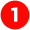Indicates the system namespace.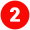Indicates the custom user defined namespace. It can be changed and it’s created once we create the c# project.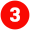Class name of the program which we’ve created. In our class, the class name is Program.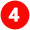All programs will start executing from a standard starting point that’s static void Main(string[] args) function.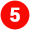For each loop to iterate the integer object returned by the method GetRandomNumbers. The program expects 20 random number which is passed as count to             the function. foreach (int i in GetRandomNumbers(20))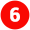Function call with count as the parameter and an array of integer which returned as list.
public static List<int> GetRandomNumbers(int count)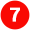Initializing the randomNumbers list variable with the code
List<int> randomNumbers = new List<int>();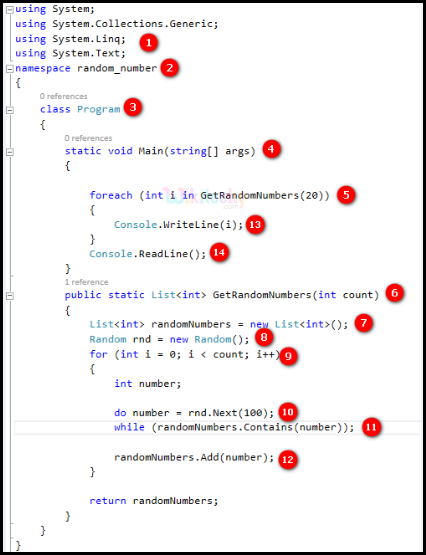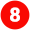Random function is called to initialize the object rnd.   Random rnd = new Random();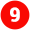Looping through a for loop upto count value. for (int i = 0; i < count; i++)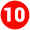Random number is assigned to the variable number.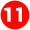The number is validated with the existing randomNumber list variable. If it’s there, loop through once again or else add  it to the randomNumber list variable.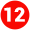Add the value into the randomNumber list variable.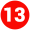Print the values from the foreach loop in the console.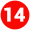This makes us to hold the output window to see the output. Waiting for the user input is the exact meaning of the statement.
Output :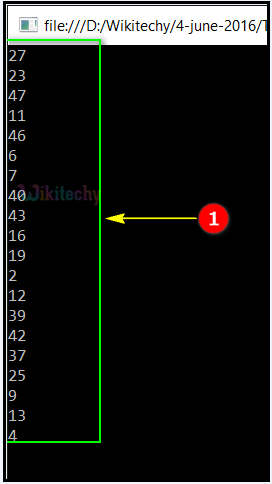Hence the output of 20 random numbers with out duplicates in c# has been executed successfully.

Bug Bounty
IOT Hackathon
Webinar